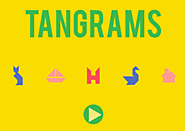Updated by Grade 3 ISA on Mar 15, 2018
REPORTGrade 3 ISA
Owner
18 items   5 followers   0 votes   332 views

# Math - Geometry

1

## Congruent & Similar Shapes - Geometry: Math Games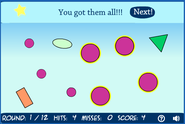Learn about triangles the fun way in this Triangle Shoot Geometry Math Game.

2

## Solid Figure Factory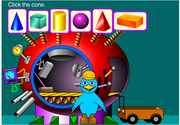3

## Solid Figures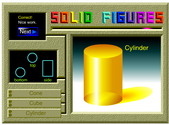4

## shape3da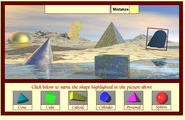5

## BBC - Starship - Maths - Calamity's Quest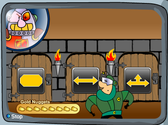6

## BBC - KS1 Bitesize Maths - Shape Lab : Fullscreen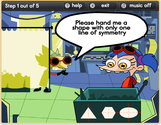A fun activity to help children at KS1 learn about 2D and 3D shapes.

7

## en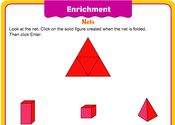8

## Shapes Shoot Math Game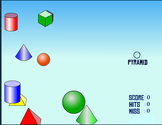Learn basic flat and solid shapes with this fun math game.

9

## Symmetry Shapes - Geometry: Math Games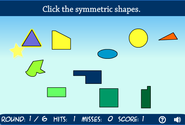Learn about symmetry the fun way in this Symmetry Shoot Geometry Math Game.

10

## Lines of Symmetry - Geometry: Math Games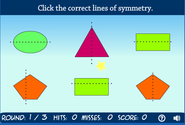Learn about lines of symmetry the fun way in this Shape Shoot Geometry Math Game.

11

## Complete the Symmetry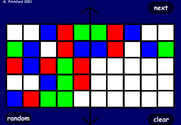12

## Sort the Symmetry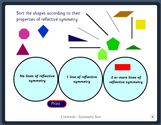Free Cool Maths kids Games. Interactive, Primary or Elementary, Key Stage 2, Ages 7-11 Years, Numeracy and Maths Shape and Weight Teacher Resources and Activities. Ideal for Helping Your Child with Maths. Use on an IWB in the Classroom, or a PC and Mac. Perfect to use for a homeschooling maths lesson.

13

## Symmetry Games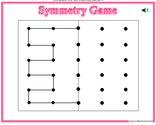Symmetry Games and Symmetry Activities: Complete the symmetrical pattern.

14

## BBC - KS2 Bitesize Maths - Symmetry : PlayMaths shape and space exercise - Symmetry activity

15

## BBC - KS2 Bitesize Maths - 3D shapes : Play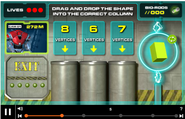Maths shape and space exercise - 3D shapes activity

16

## Math - Geometry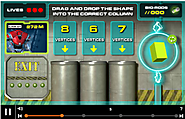Math - Geometry | Congruent & Similar Shapes - Geometry: Math Games, Solid Figure Factory, Solid Figures, shape3da, BBC - Starship - Maths - Calamity's Quest, BBC - KS1 Bitesize Maths - Shape Lab : Fullscreen, en, Shapes Shoot Math Game, Symmetry Shapes - Geometry: Math Games, and Lines of Symmetry - Geometry: Math Games

17

## Geometry 3TW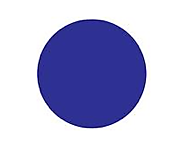Vocabulary words for Geometry 3TW. Includes studying games and tools such as flashcards.

18

## ABCya! Tangrams